# Converting between Scientific Notation & Standard Form Lesson Plan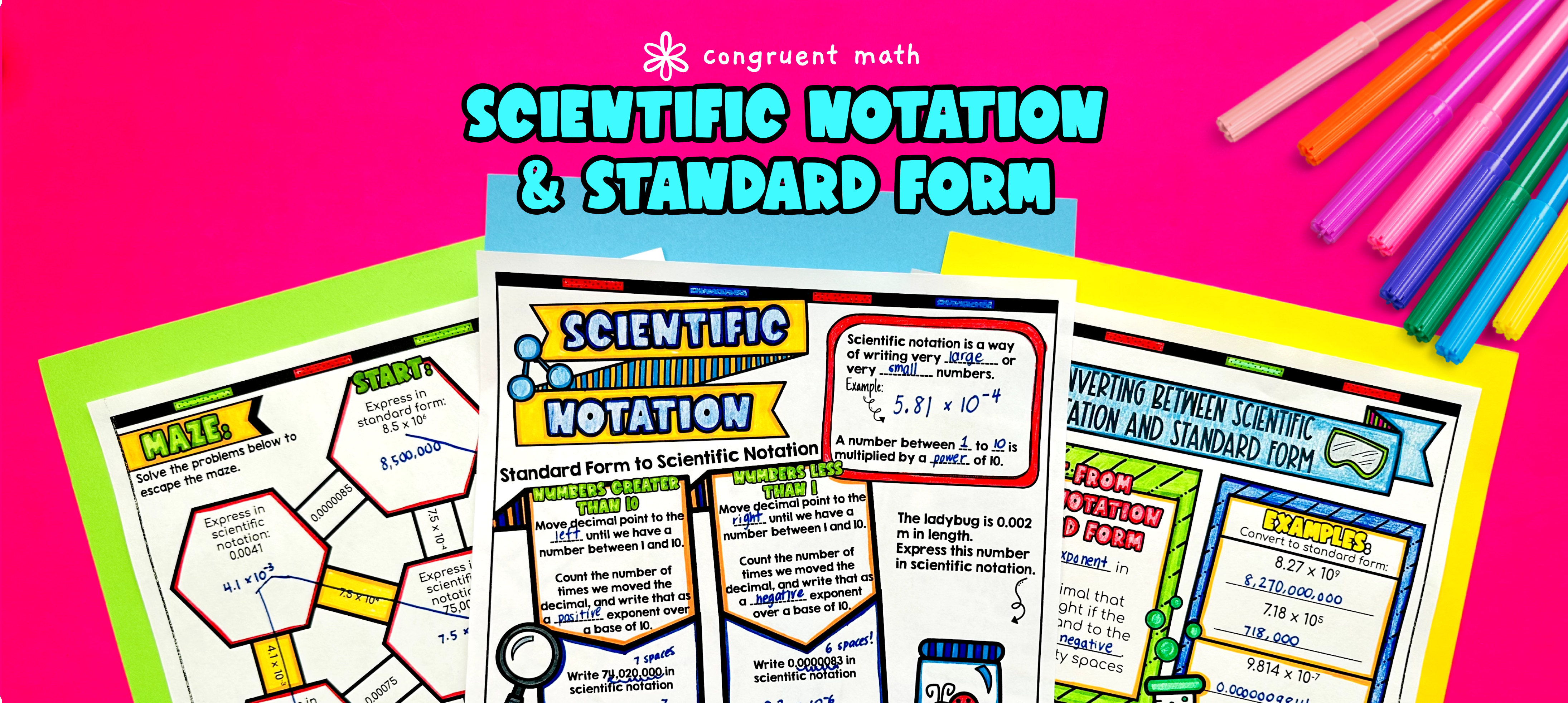Pin This

## Overview

Ever wondered how to teach scientific notation in an engaging way to your eighth-grade students?

In this lesson plan, students will learn about scientific notations and their real-life applications. Through artistic, interactive guided notes, check for understanding, a doodle & color by number activity, and a maze worksheet, students will gain a comprehensive understanding of converting between standard form and scientific notations. They will learn the step-by-step process and practice with various examples and exercises.

The lesson concludes with a detailed real life example that explores how scientific notation is used in real-world scenarios. Students will read and write about the practical applications of expressing numbers in scientific notations.

## Get the Lesson Materials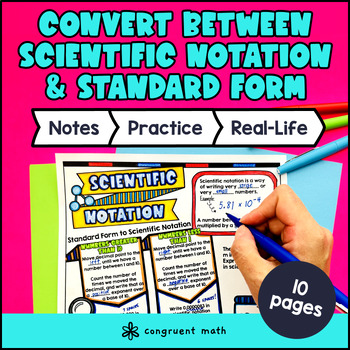\$4.25

## Learning Objectives

After this lesson, students will be able to:

• Convert numbers from scientific notation to standard form and vice versa

• Apply scientific notation to real-life situations to represent large and small numbers

• Explain the significance and benefits of using scientific notation in scientific in real life

## Prerequisites

Before this lesson, students should be familiar with:

• Knowledge of place value and the concept of decimal numbers

## Key Vocabulary

• Scientific notation

• Standard form

• Exponent

• Base

• Power of ten

## Procedure

### Introduction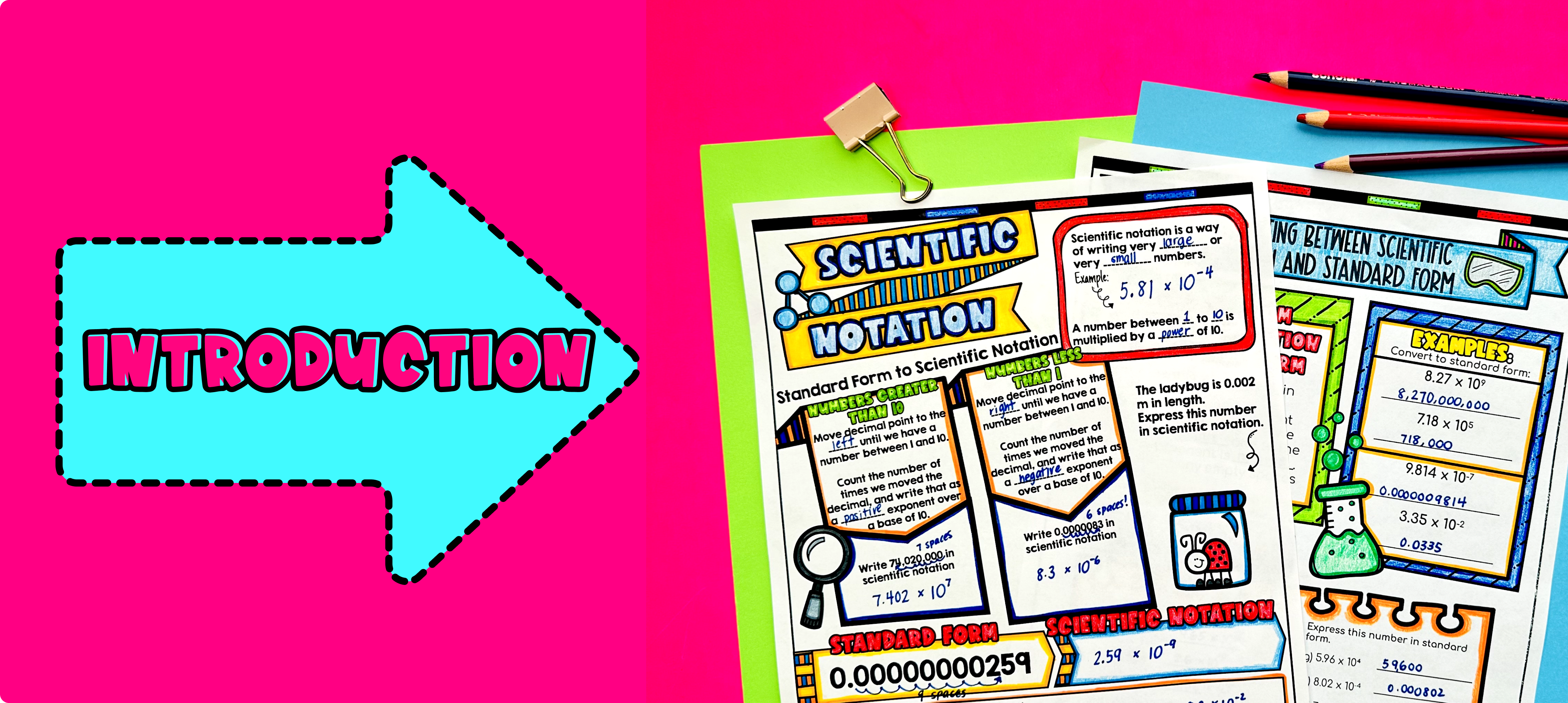Pin This

As a hook, ask students why it is important to be able to express numbers in scientific notation. Refer to the last page of the guided notes, where students can read and write about real-life uses of expressing numbers in scientific notations. This will help students see the relevance and importance of the topic.

Use the first two pages of the guided notes to introduce the concept of scientific notation. Walk through the topic's key points, such as what scientific notation is, why it is used, and how to convert numbers between standard form and scientific notation.

Based on student responses, reteach any concepts that students need extra help with, especially in understanding the basic principles of scientific notation. If your class has a wide range of proficiency levels, you can pull out students for reteaching, and have more advanced students begin work on the practice exercises.

### Practice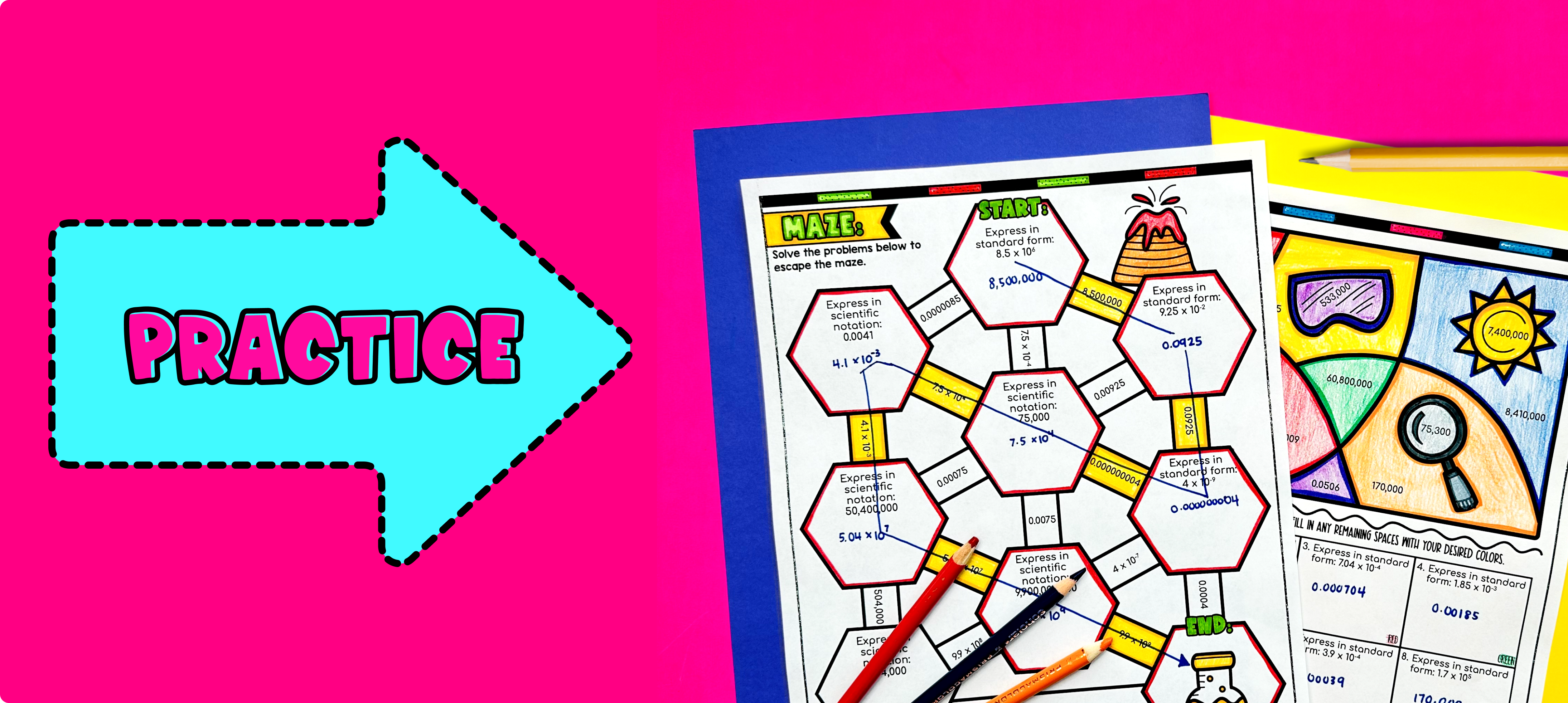Pin This

Have students practice converting between standard form and scientific notation using the practice sections at the bottom of the guided notes as well as the maze activity (page 3 of guided notes). Walk around the classroom to answer any student questions and provide guidance as needed.

Fast finishers can then move on to the color by number activity worksheet for additional practice (page 4 of guided notes). You can assign this maze activity as homework for students to complete for the remainder of the class.

### Real-Life Application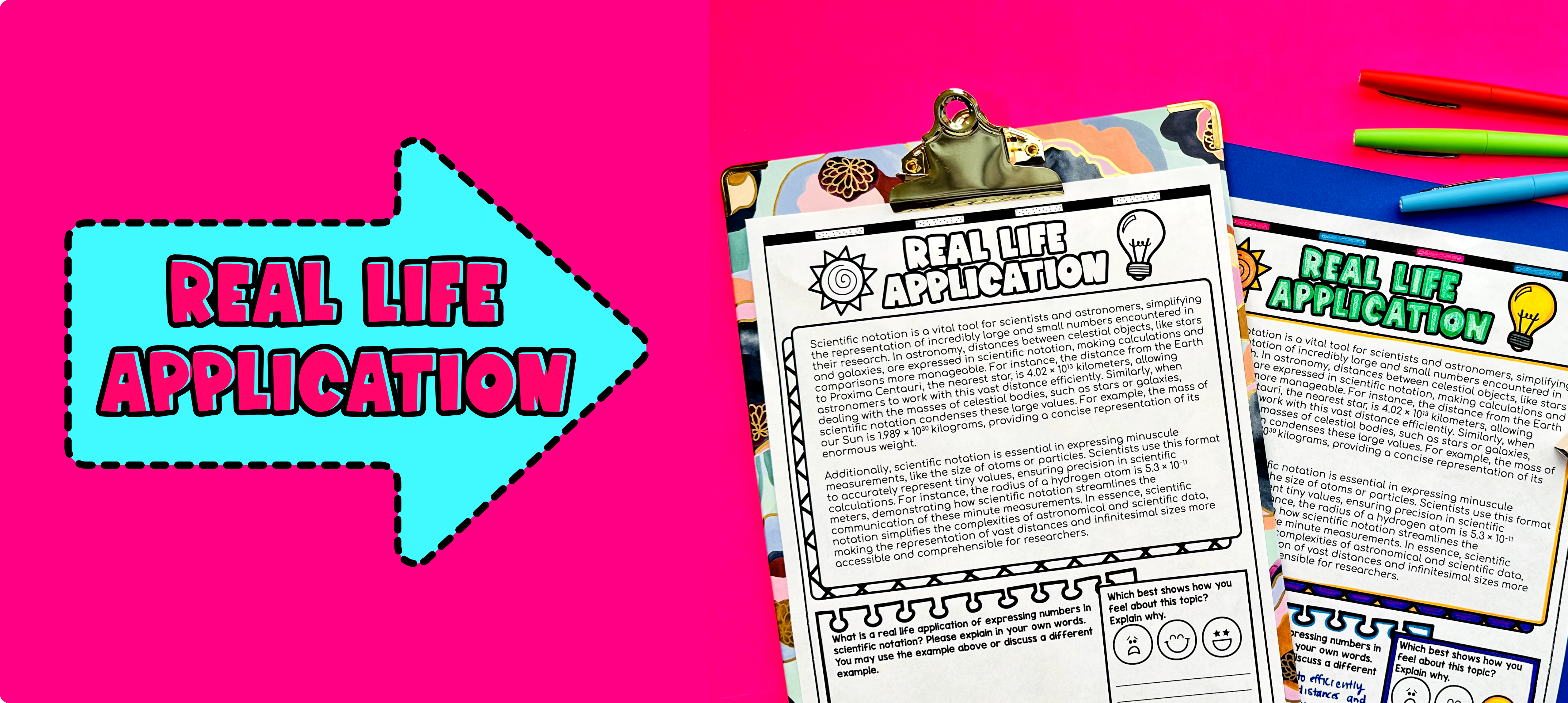Pin This

Bring the class back together, and introduce the concept of real-world applications of expressing numbers in scientific notations. Use the last page of the guided notes to allow students to read about a detailed example of scientific notation in real life.

Explain to the students that scientific notation is commonly used in scientific fields such as astronomy, physics, and chemistry to represent extremely large or small numbers more conveniently.

To further illustrate the real-life application of scientific notation, provide examples such as:

1. Astronomy: Explain that scientists often use scientific notation to represent distances, such as the distance between stars and galaxies. For example, the distance between the Earth and the Sun is approximately 93 million miles, which can be written as 9.3 x 10^7 miles in scientific notation. This allows scientists to work with and compare distances more easily.

2. Medicine: Discuss how scientists and doctors may use scientific notation to express very small measurements, such as the size of bacteria or viruses. For instance, the size of a virus can be written as 2.1 x 10^-9 meters. This method avoids writing long decimal numbers and simplifies calculations.

3. Financial Transactions: Mention that financial institutions often use scientific notation when dealing with large amounts of money. For example, when discussing national debts or the gross domestic product (GDP) of a country, numbers can be expressed in scientific notation to make them more manageable. For instance, the national debt of the United States is approximately \$28 trillion, which can be written as 2.8 x 10^13 dollars.

Encourage students to think of other real-life examples where expressing numbers in scientific notation could be useful.

## Extensions

If you're looking for digital practice for converting between standard form and scientific notation, try my Pixel Art activities in Google Sheets. Every answer is automatically checked, and correct answers unlock parts of a mystery picture. It's incredibly fun and a powerful tool for differentiation.

Here are some activities to explore: